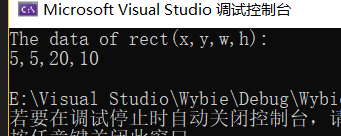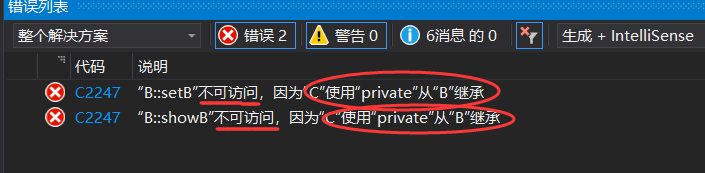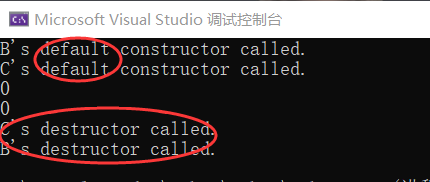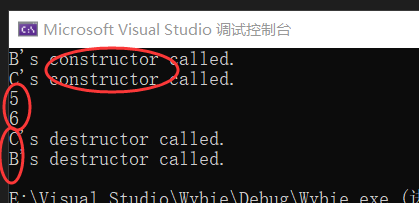# 继承与派生

## 二、访问控制

### 公有继承

#include<iostream>
#include<cmath>
using namespace std;
class Point {    //基类：点类
public:
void initPoint(float x = 0, float y = 0) { this->x = x; this->y=y; }
void move(float offX, float offY) { x += offX; y += offY; }
float getX()const { return x; }
float getY()const { return y; }
private:
float x, y;
};
class Rectangle :public Point {  //点类的派生类，对其公有继承
public:
void initRectangle(float x, float y, float w, float h) {
initPoint(x, y);         //公有继承，可以使用基类的成员
this->w = w;
this->h = h;
}
float getH()const { return h; }
float getW()const { return w; }
private:
float w, h;
};
int main() {
Rectangle rect;
rect.initRectangle(2, 3, 20, 10);
rect.move(3, 2);
cout << "The data of rect(x,y,w,h):" << endl;
cout << rect.getX() << ","
<< rect.getY() << ","
<< rect.getW() << ","
<< rect.getH() << endl;
return 0;
}### 私有继承

//header.h
class A {
public:
void setA(int);
void showA()const;
private:
int a;
};
class B {
public:
void setB(int);
void showB()const;
private:
int b;
};
class C :public A, private B {
public:
void setC(int, int, int);
void showC()const;
private:
int c;
};

//caroline.cpp
#include<iostream>
using namespace std;
void A::setA(int x) {
a = x;
}
void A::showA()const {
cout << a << endl;
}
void B::setB(int x) {
b = x;
}
void B::showB()const {
cout << b << endl;
}
void C::setC(int x, int t, int z) {
setA(x);
setB(y);
c = z;
}
void C::showC const() {
cout << c << endl;
}

//wybie.cpp
#include<iostream>
using namespace std;
int main() {
C obj;
obj.setA(5);
obj.showA();
obj.setC(6,7,9);
obj.showC();
obj.setB(6);
obj.showB();
return 0;
}### 保护继承

//基类A：
class A{
protected:
int x;
};
//主函数：
int main(){
A a;
a.x=5;
}


class A{
protected :
int x;
};
calss B: public A{
public:
void function();
};
void B::function(){
x=5;
}
int main(){
B b;
b.function();
return 0;
}


## 三、派生类的构造函数和析构函数

派生类名::派生类名（参数表）：基类名1（基类1初始化参数表），、、、，基类名n（基类n初始化参数表），成员对象名1（成员对象1初始化参数表），、、、，成员对象名m（成员对象m初始化参数表）
{
派生类构造函数的其他初始化操作
}


1. 调用基类构造函数，调用顺序按照它们被继承时声明的顺序（从左向右）。
2. 执行派生类的构造函数体中的内容。

#include<iostream>
using namespace std;
class B {
public:
B();  //默认构造函数
B(int i);  //有参数的构造函数
~B();     //析构函数
void print()const;
private:
int b;
};
B::B()
{
b = 0;
cout << "B's default constructor called." << endl;
}
B::B(int i) {
b = i;
cout << "B's constructor called." << endl;
}
B::~B() {
cout << "B's destructor called." << endl;
}
void B::print()const
{
cout << b << endl;
}
class C :public B {
public:
C();    //默认构造函数
C(int i, int j);  //有参数
~C();             //C的析构函数
void print()const;
private:
int c;
};
C::C() {
c = 0;
cout << "C's default constructor called." << endl;
}
C::C(int i, int j):B(i),c(j) {
cout << "C's destructor called." << endl;
}
C::~C() {
cout << "C's destructor called." << endl;
}
void C::print()const
{
B::print();
cout << c << endl;
}
int main() {
C obj;   //不带参数，自动调用B::B()、C::C()
obj.print();
return 0;
}int main() {
C obj(5, 6);  //对象有两个参数，自动调用C::C(5,6),进而首先调用B::B(5),然后执行C::C(5，6)的初始化列表和函数体
obj.print();
return 0;
}posted @ 2019-10-12 10:52  Wybie  阅读(283)  评论(0编辑  收藏  举报